Learn all Concepts of Chapter 3 Class 10 (with VIDEOS). Check - Linear Equations in 2 Variables - Class 10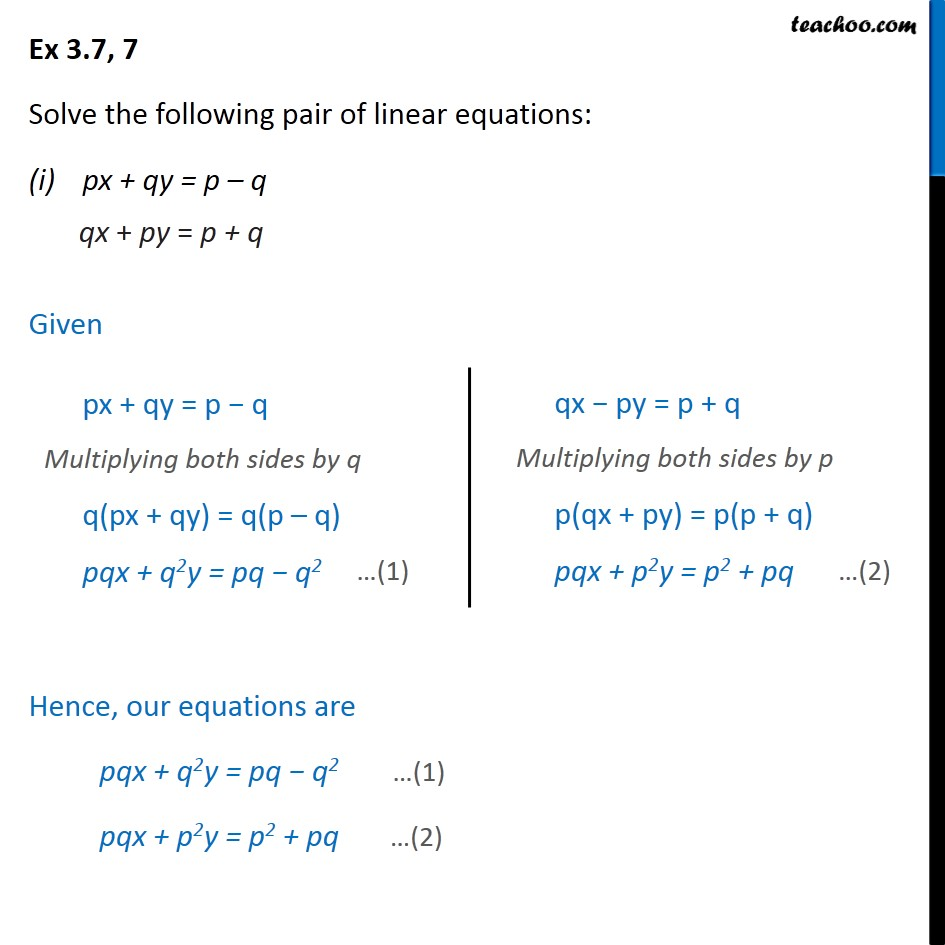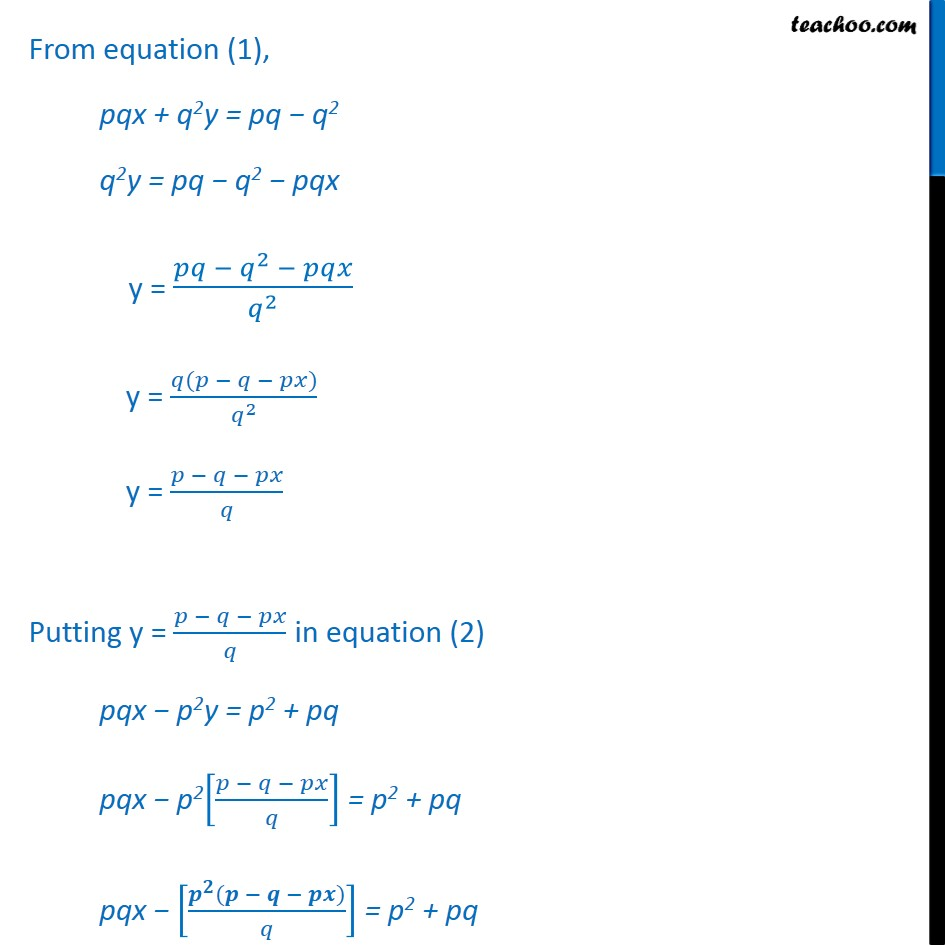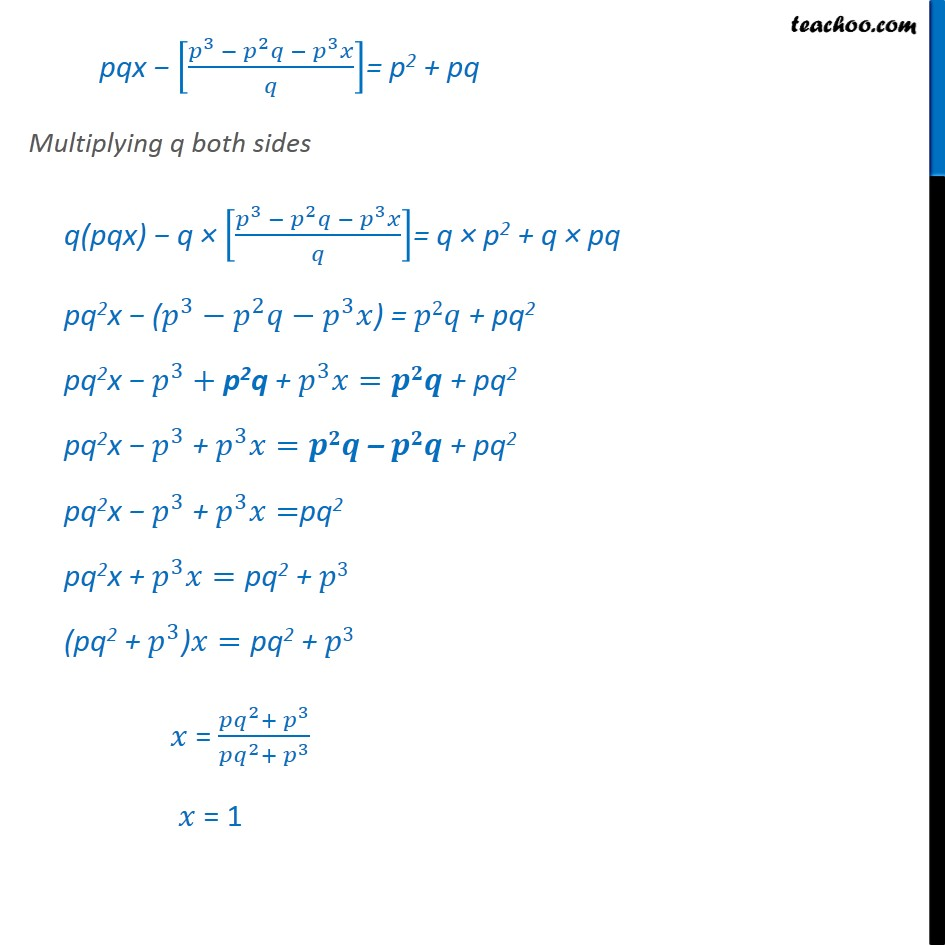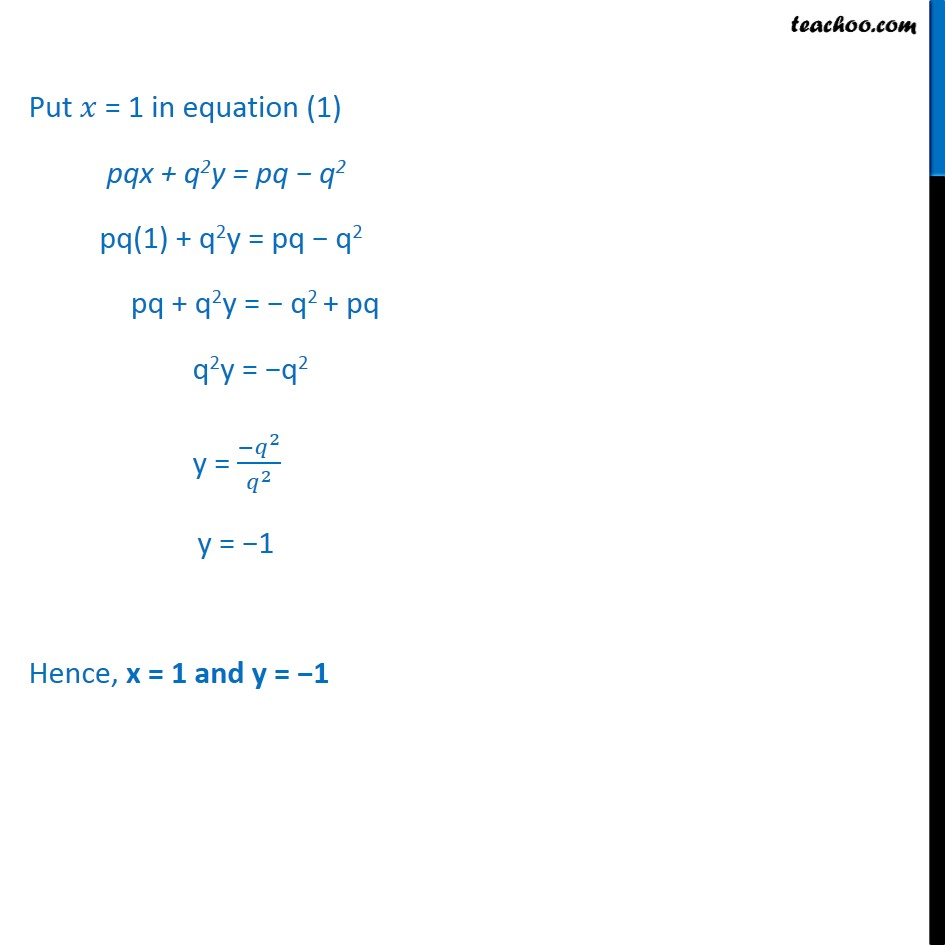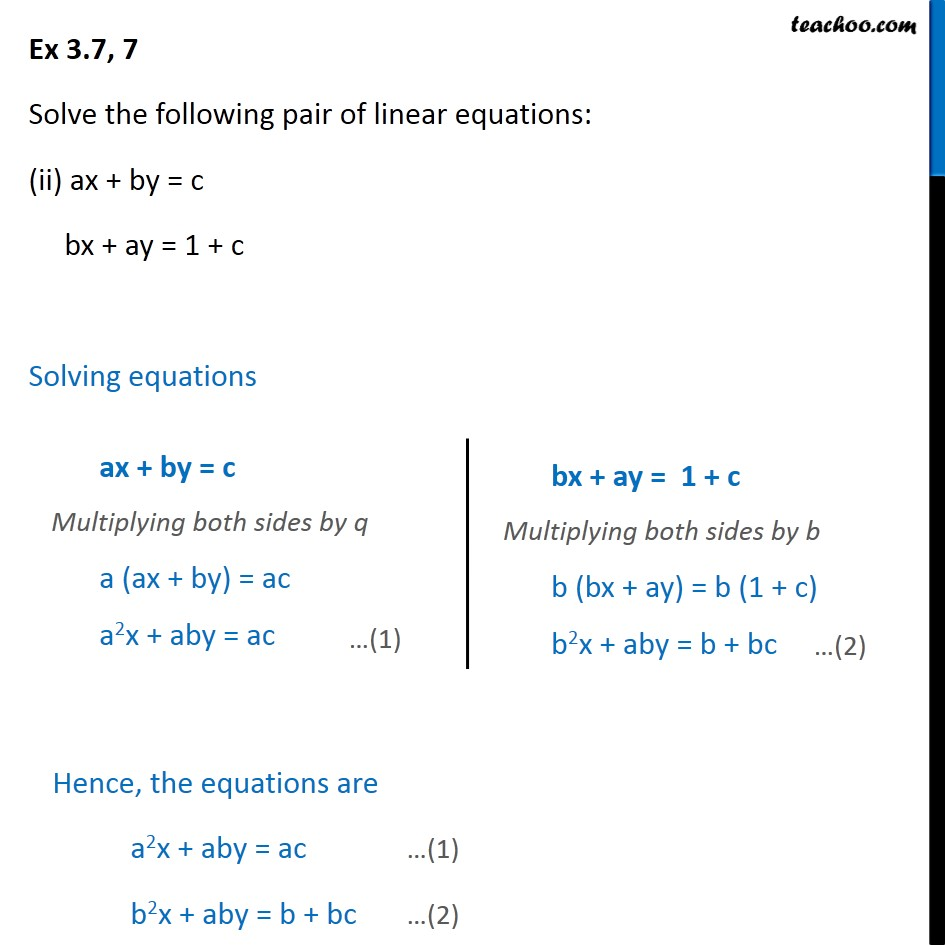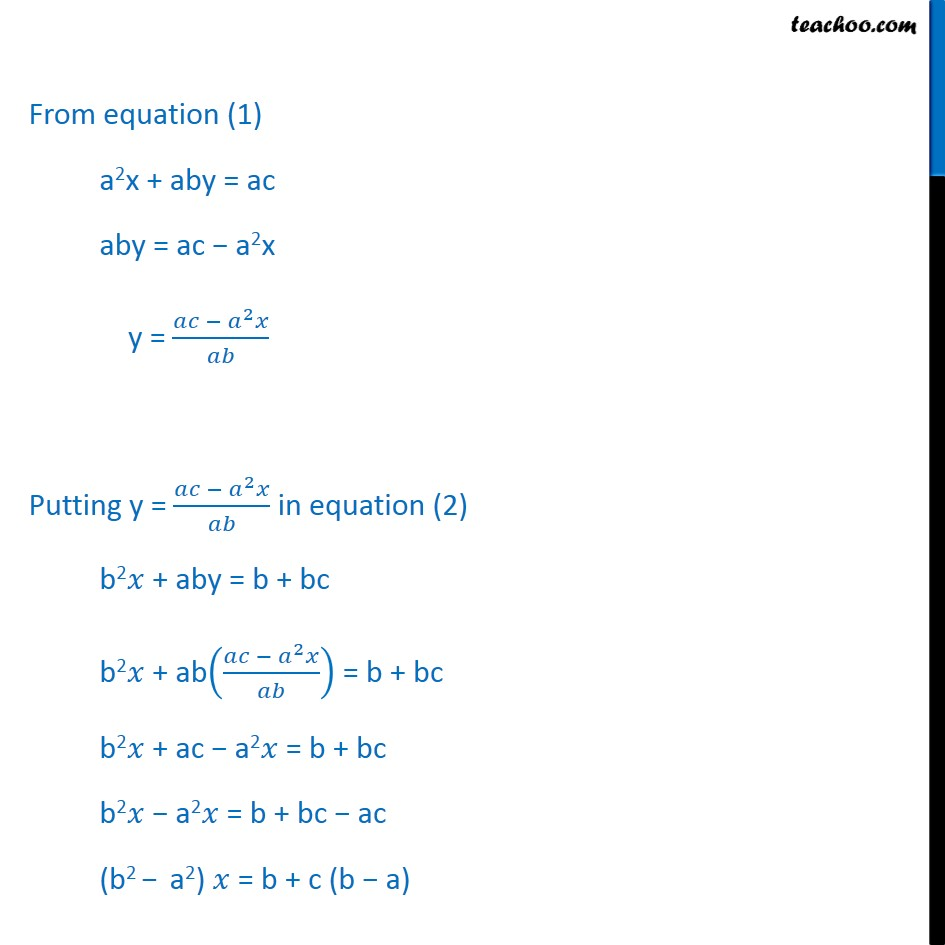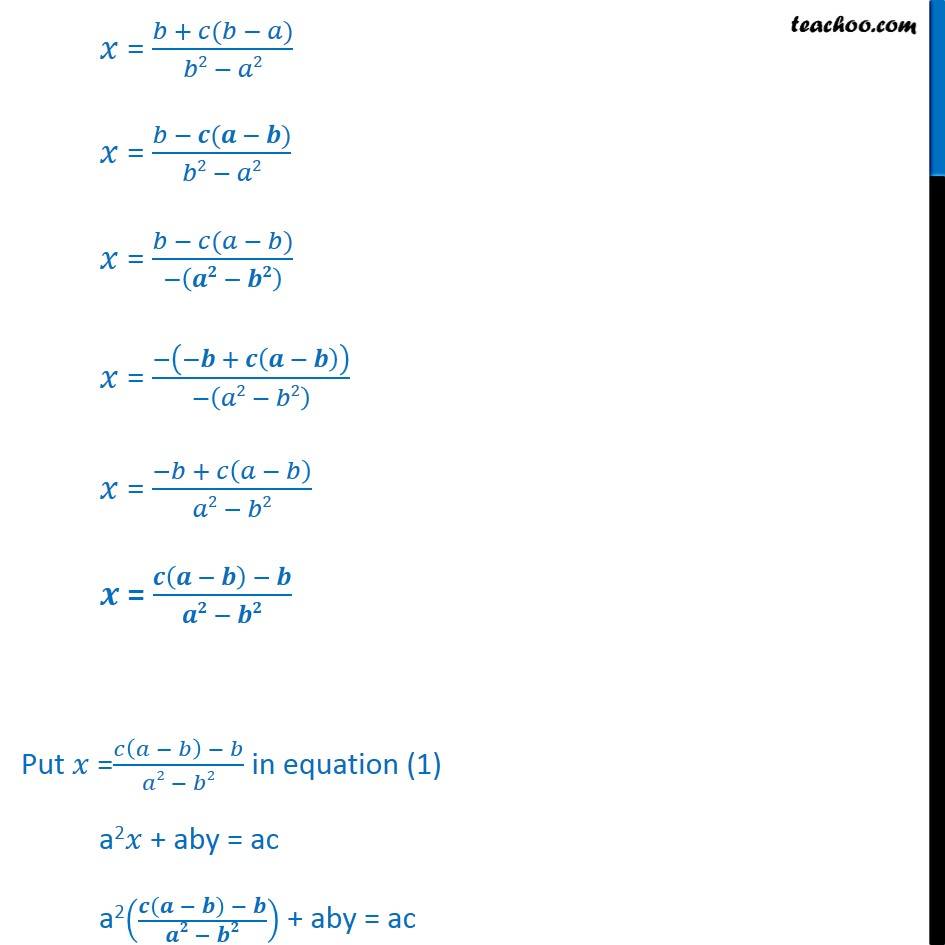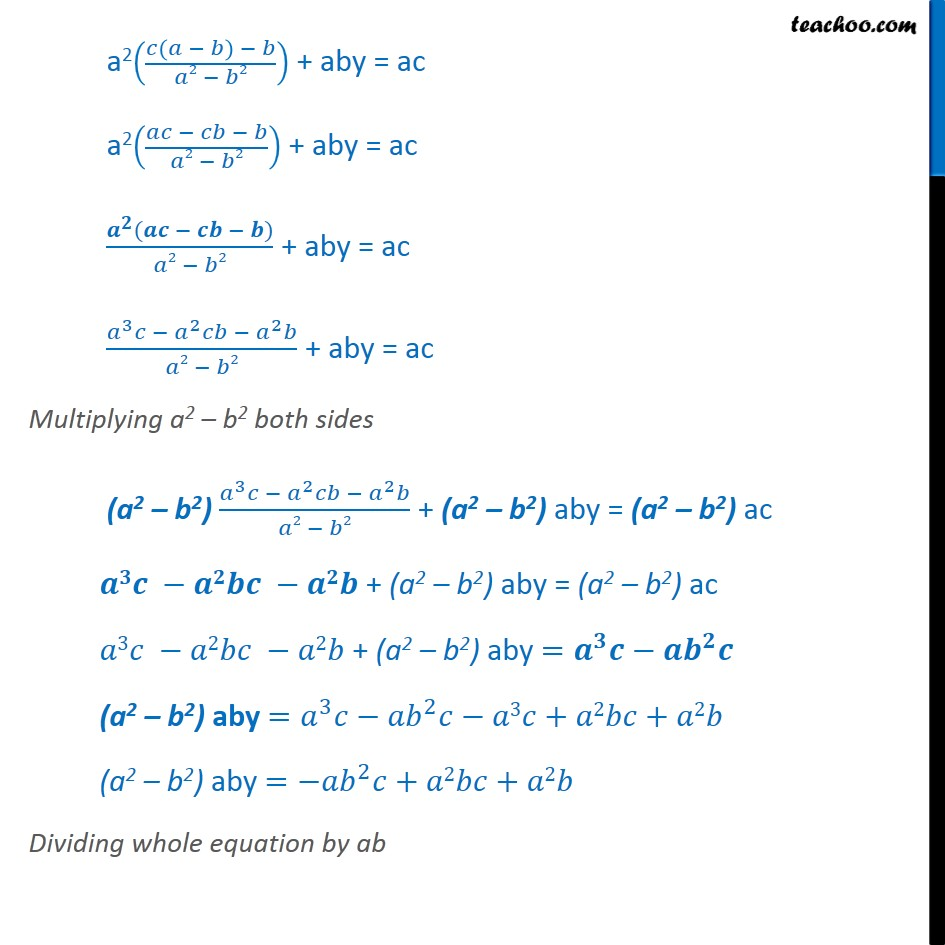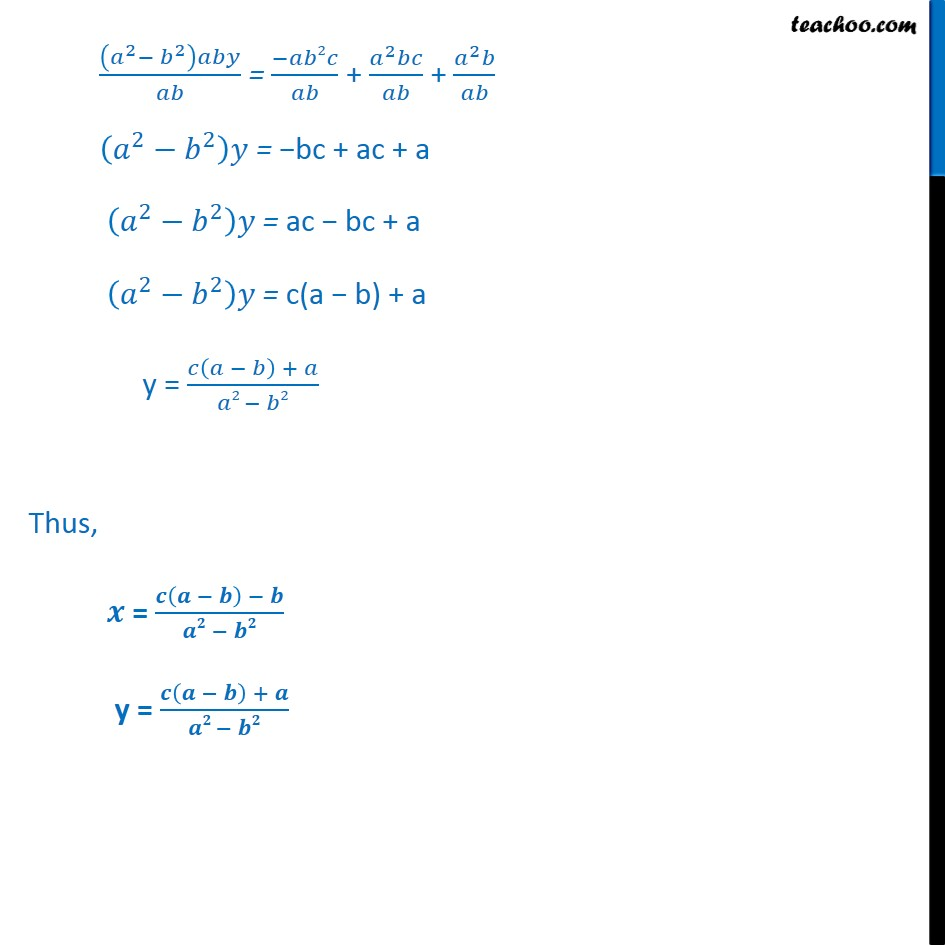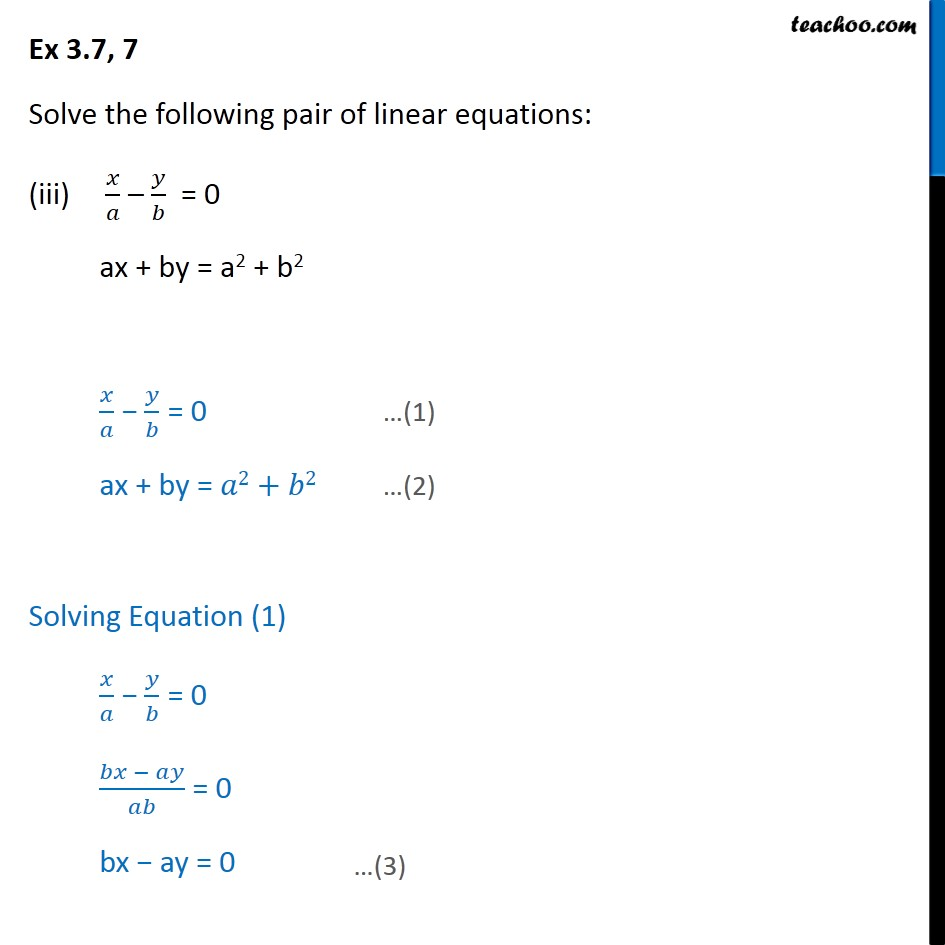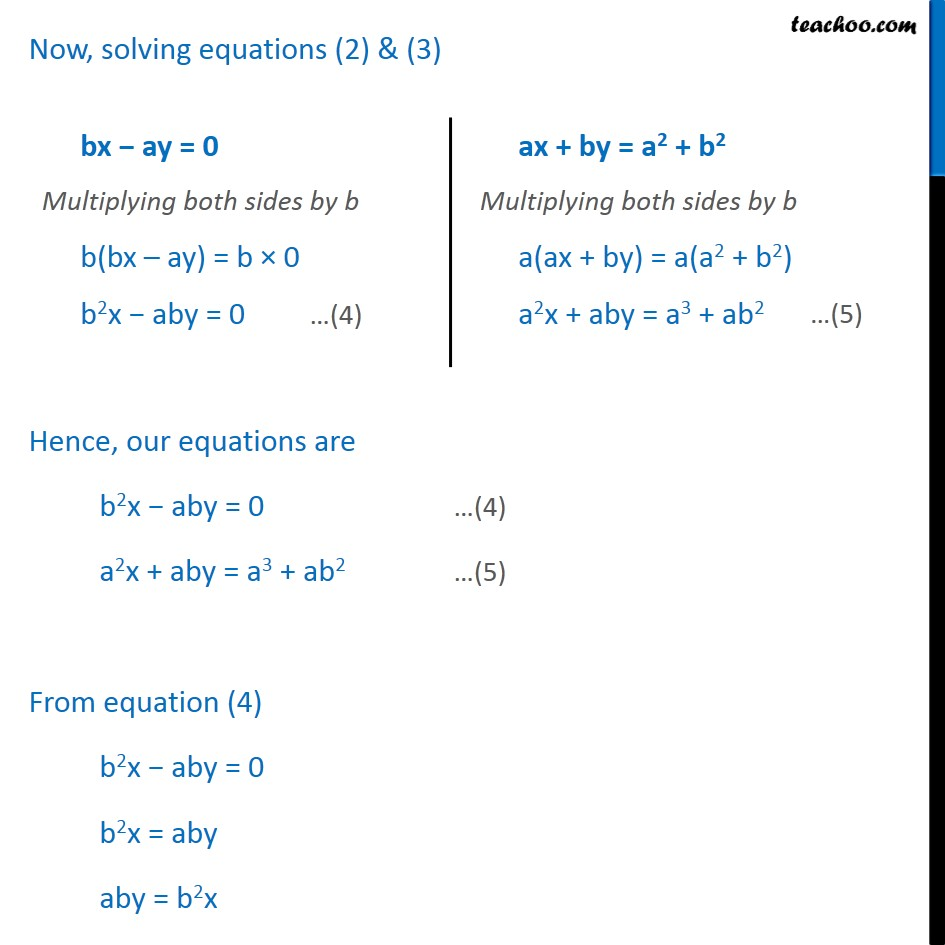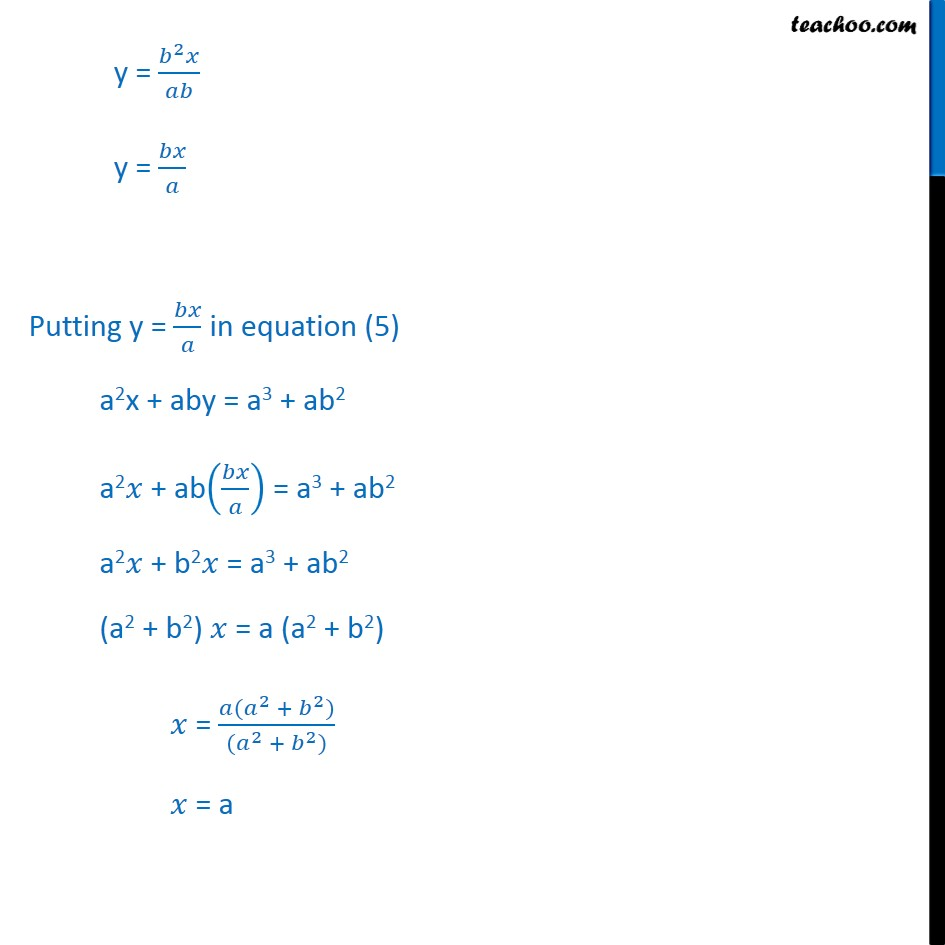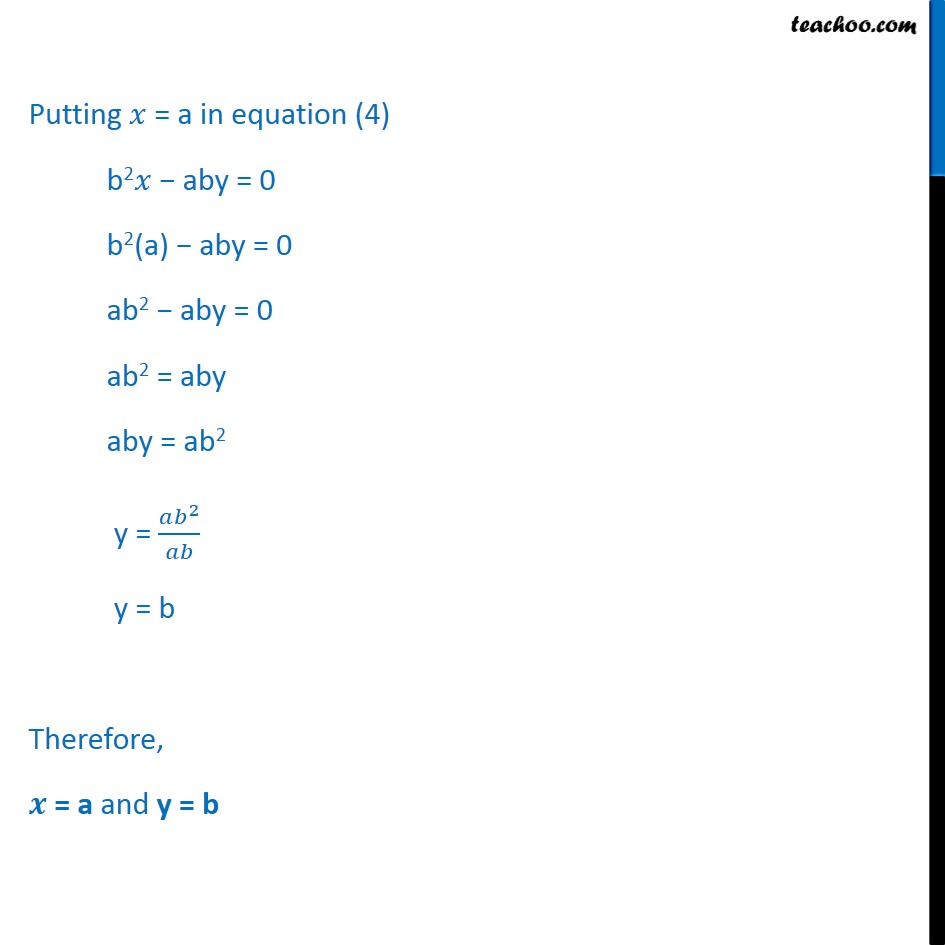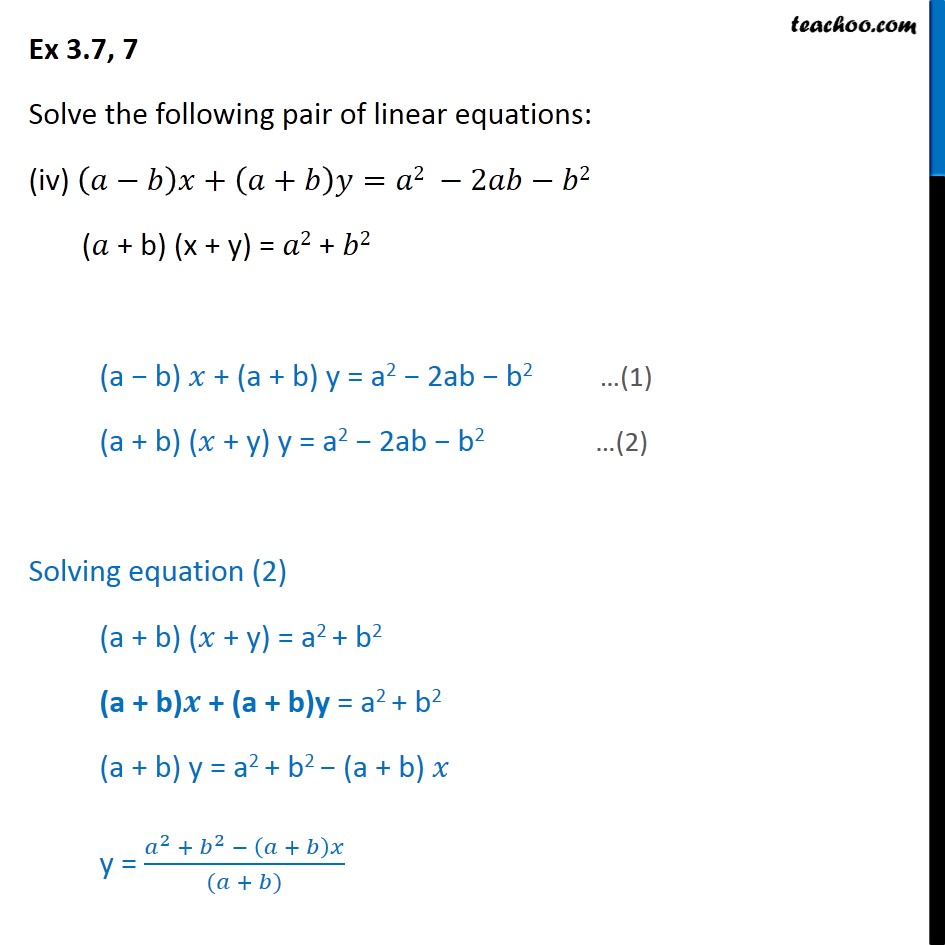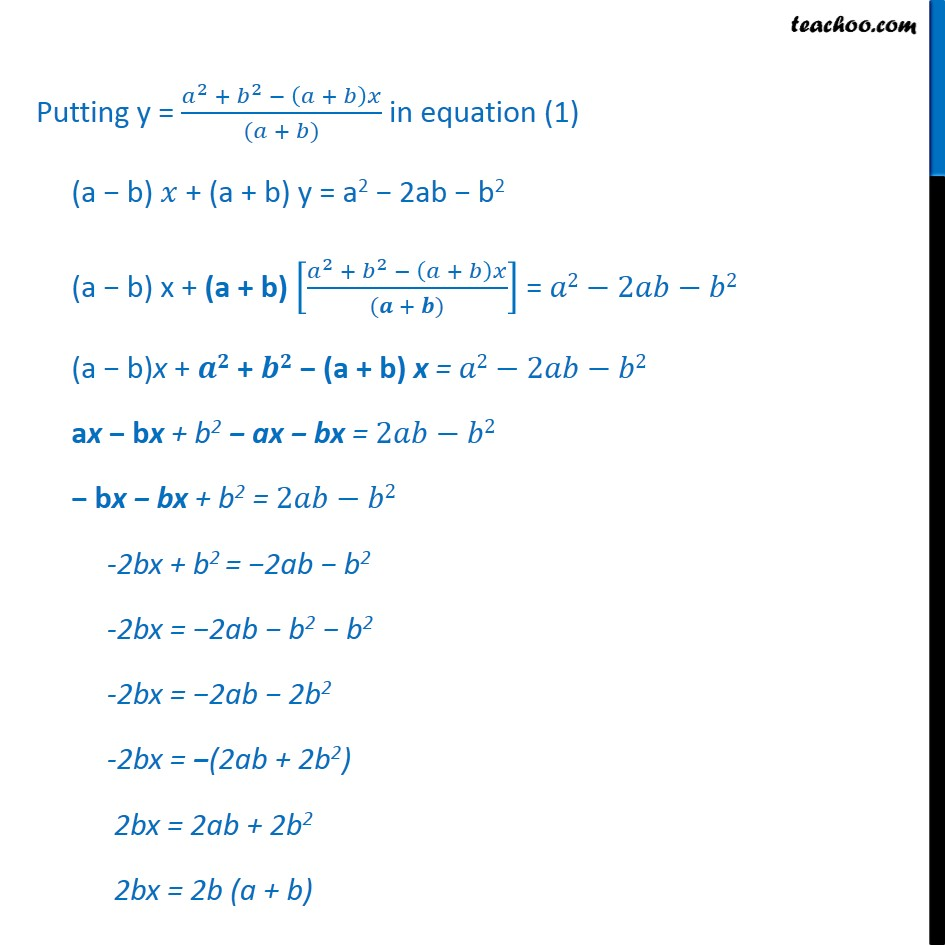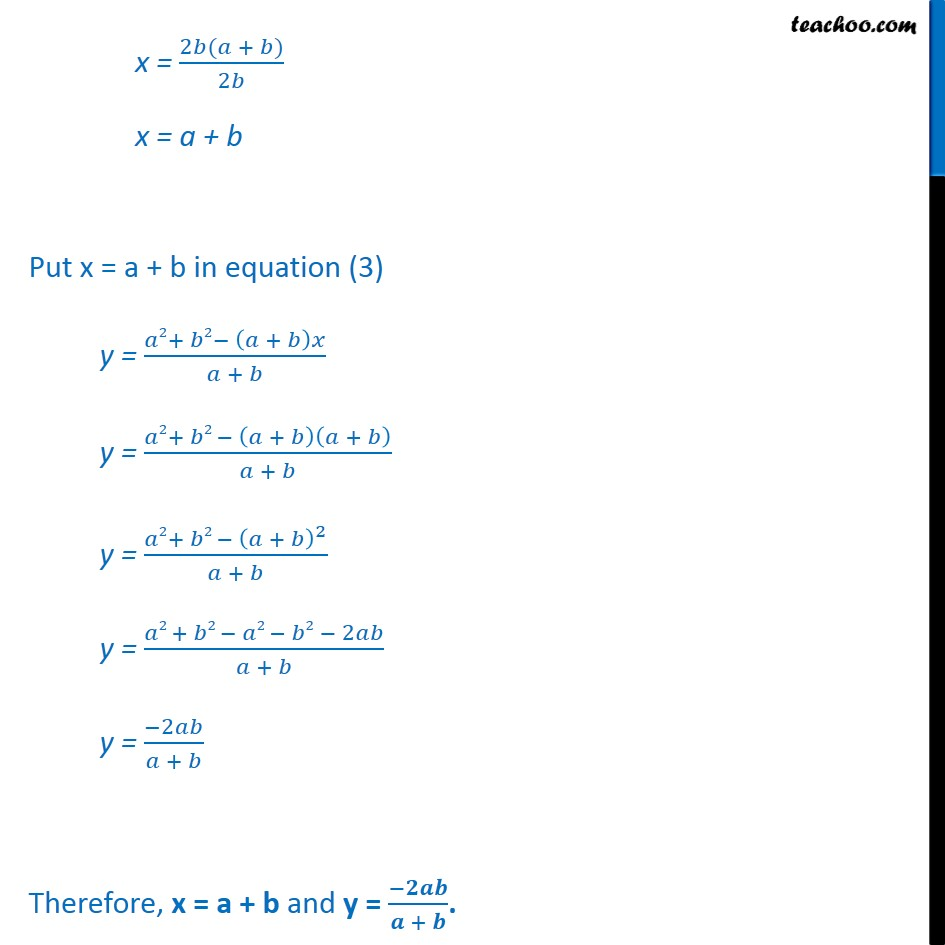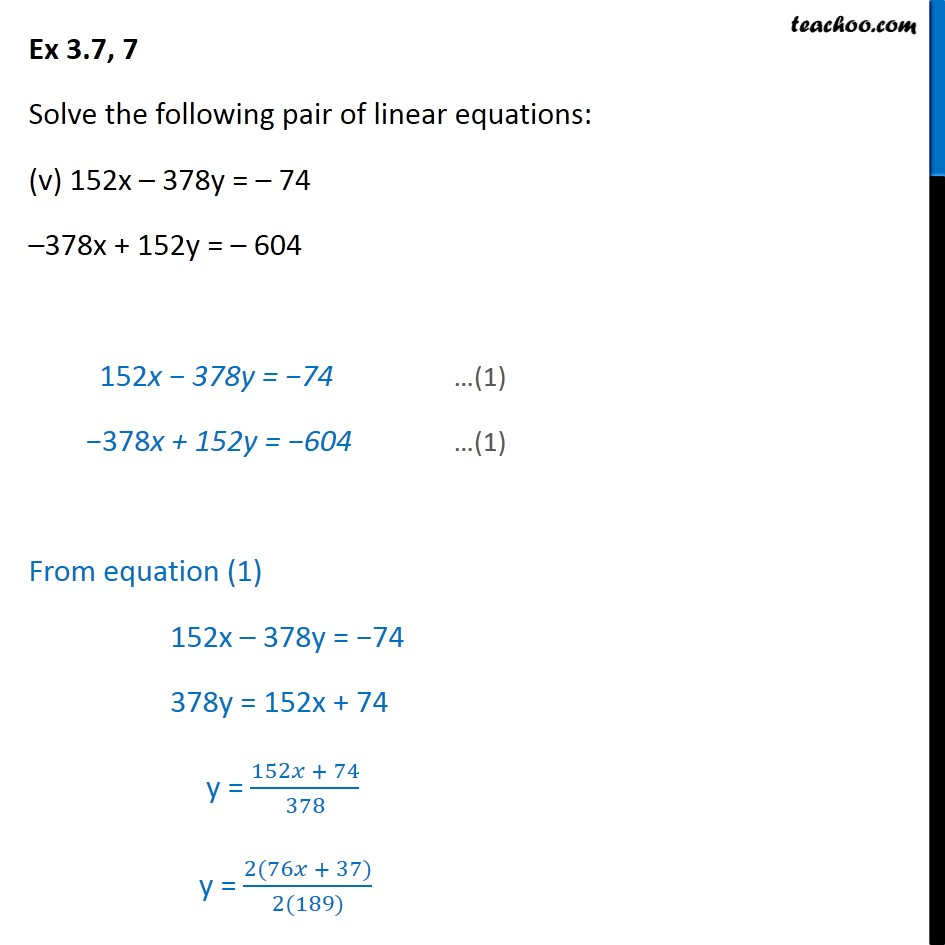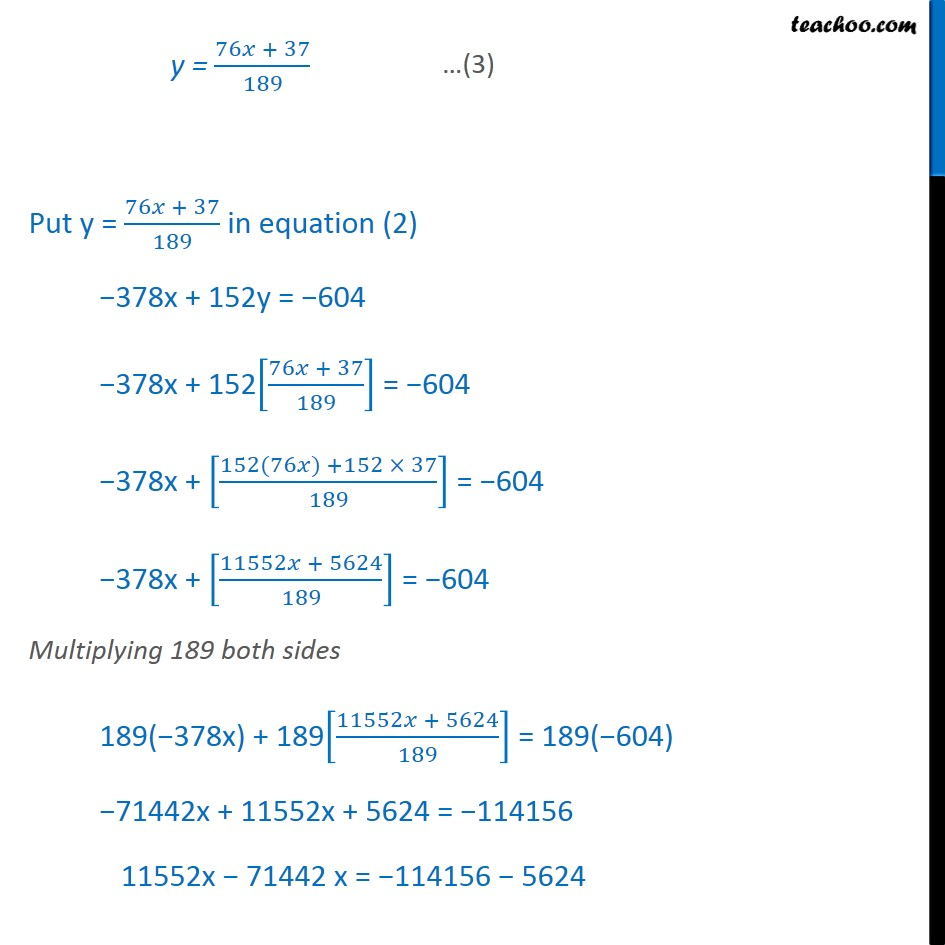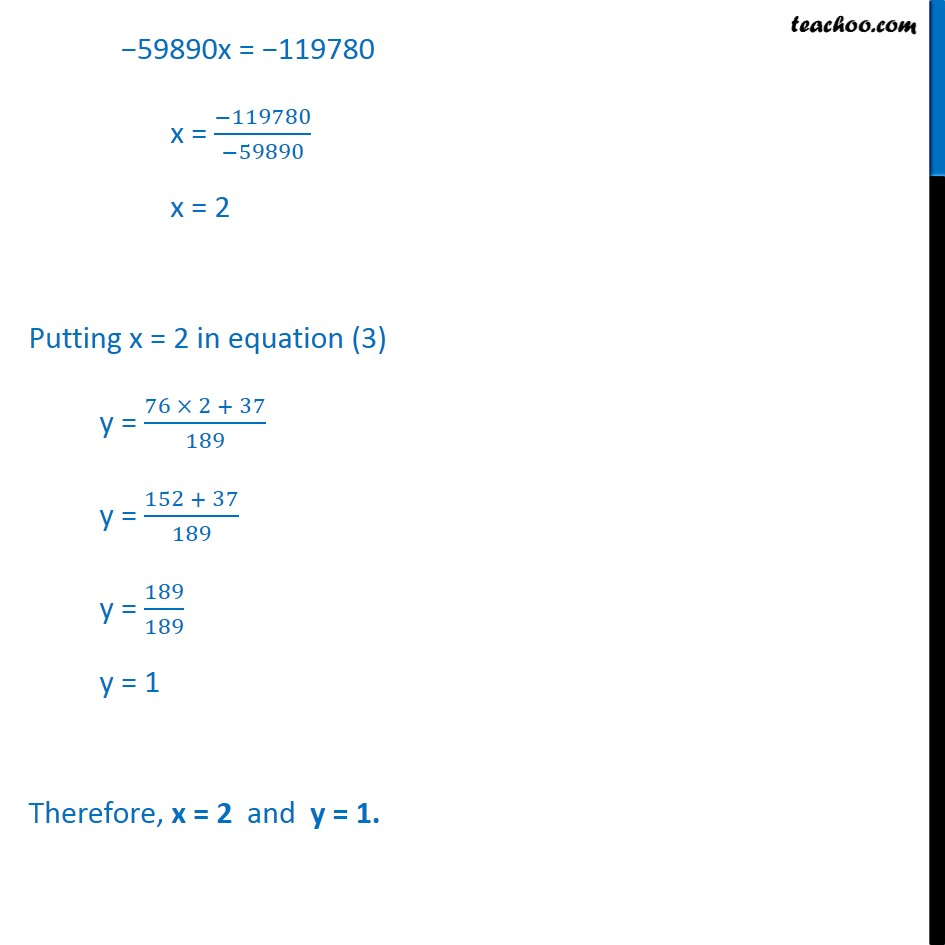1. Chapter 3 Class 10 Pair of Linear Equations in Two Variables
2. Serial order wise
3. Ex.3.7 (Optional)

Transcript

Ex 3.7, 7 Solve the following pair of linear equations: px + qy = p q qx + py = p + q Given Hence, our equations are pqx + q2y = pq q2 (1) pqx + p2y = p2 + pq (2) px + qy = p q Multiplying both sides by q q(px + qy) = q(p q) pqx + q2y = pq q2 qx py = p + q Multiplying both sides by p p(qx + py) = p(p + q) pqx + p2y = p2 + pq From equation (1), pqx + q2y = pq q2 q2y = pq q2 pqx y = ( ^2 )/ ^2 y = ( ( ))/ ^2 y = ( )/ Putting y = ( )/ in equation (2) pqx p2y = p2 + pq pqx p2[( )/ ] = p2 + pq pqx [( ^ ( ))/ ] = p2 + pq pqx [( ^3 ^2 ^3 )/ ]= p2 + pq Multiplying q both sides q(pqx) q [( ^3 ^2 ^3 )/ ]= q p2 + q pq pq2x ( ^3 ^2 ^3 ) = 2 + pq2 pq2x ^3+"p2q + " ^3 = + pq2 pq2x ^3 " + " ^3 = + pq2 pq2x ^3 " + " ^3 =pq2 "pq2x + " ^3 ="pq2 "+ 3 "(pq2 + " ^3 ")" ="pq2 "+ 3 = ( ^2+ ^3)/( ^2+ ^3 ) = 1 Put = 1 in equation (1) pqx + q2y = pq q2 pq(1) + q2y = pq q2 pq + q2y = q2 + pq q2y = q2 y = ( ^2)/ ^2 y = 1 Hence, x = 1 and y = 1 Ex 3.7, 7 Solve the following pair of linear equations: (ii) ax + by = c bx + ay = 1 + c Solving equations ax + by = c Multiplying both sides by q a (ax + by) = ac a2x + aby = ac bx + ay = 1 + c Multiplying both sides by b b (bx + ay) = b (1 + c) b2x + aby = b + bc Hence, the equations are a2x + aby = ac (1) b2x + aby = b + bc (2) From equation (1) a2x + aby = ac aby = ac a2x y = ( ^2 )/ Putting y = ( ^2 )/ in equation (2) b2 + aby = b + bc b2 + ab(( ^2 )/ ) = b + bc b2 + ac a2 = b + bc b2 a2 = b + bc ac (b2 a2) = b + c (b a) = ( + ( ))/( 2 2) = ( ( ))/( 2 2) = ( ( ))/( ( ) ) = ( ( + ( )))/( ( 2 2) ) = ( + ( ))/( 2 2) = ( ( ) )/( ) Put =( ( ) )/( 2 2) in equation (1) a2 + aby = ac a2(( ( ) )/( )) + aby = ac a2(( ( ) )/( )) + aby = ac a2(( ( ) )/( 2 2)) + aby = ac a2(( )/( 2 2)) + aby = ac ( ^ ( ))/( 2 2) + aby = ac ( ^3 ^2 ^2 )/( 2 2) + aby = ac Multiplying a2 b2 both sides (a2 b2) ( ^3 ^2 ^2 )/( 2 2) + (a2 b2) aby = (a2 b2) ac + (a2 b2) aby = (a2 b2) ac 3 2 2 + (a2 b2) aby = ^ ^ (a2 b2) aby = ^3 ^2 3 + 2 + 2 (a2 b2) aby = ^2 + 2 + 2 Dividing whole equation by ab ( ^2 ^2 ) / = ( 2 )/ + ( ^2 )/ + ( ^2 )/ ( ^2 ^2 ) = bc + ac + a " " ( ^2 ^2 ) " = ac bc + a" " " ( ^2 ^2 ) " = c(a b) + a" y = ( ( ) + )/( 2 2) Thus, = ( ( ) )/( ) y = ( ( ) + )/( ) Ex 3.7, 7 Solve the following pair of linear equations: (iii) / / = 0 ax + by = a2 + b2 / / = 0 (1) ax + by = 2+ 2 (2) Solving Equation (1) / / = 0 ( )/ = 0 bx ay = 0 bx ay = 0 Multiplying both sides by b b(bx ay) = b 0 b2x aby = 0 ax + by = a2 + b2 Multiplying both sides by b a(ax + by) = a(a2 + b2) a2x + aby = a3 + ab2 Now, solving equations (2) & (3) Hence, our equations are b2x aby = 0 (4) a2x + aby = a3 + ab2 (5) From equation (4) b2x aby = 0 b2x = aby aby = b2x y = ( ^2 )/ y = / Putting y = / in equation (5) a2x + aby = a3 + ab2 a2 + ab( / ) = a3 + ab2 a2 + b2 = a3 + ab2 (a2 + b2) = a (a2 + b2) = ( ( ^2 + ^2))/(( ^2 + ^2)) = a Putting = a in equation (4) b2 aby = 0 b2(a) aby = 0 ab2 aby = 0 ab2 = aby aby = ab2 y = ( ^2)/ y = b Therefore, = a and y = b Ex 3.7, 7 Solve the following pair of linear equations: (iv) ( ) +( + ) = 2 2 2 ( + b) (x + y) = 2 + 2 (a b) + (a + b) y = a2 2ab b2 (1) (a + b) ( + y) y = a2 2ab b2 (2) Solving equation (2) (a + b) ( + y) = a2 + b2 (a + b) + (a + b)y = a2 + b2 (a + b) y = a2 + b2 (a + b) y = ( ^2 + ^2 ( + ) )/(( + )) Putting y = ( ^2 + ^2 ( + ) )/(( + )) in equation (1) (a b) + (a + b) y = a2 2ab b2 (a b) x + (a + b) [( ^2 + ^2 ( + ) )/(( + ))] = 2 2 2 (a b)x + + (a + b) x = 2 2 2 ax bx + b2 ax bx = 2 2 bx bx + b2 = 2 2 -2bx + b2 = 2ab b2 -2bx = 2ab b2 b2 -2bx = 2ab 2b2 -2bx = (2ab + 2b2) 2bx = 2ab + 2b2 2bx = 2b (a + b) x = (2 ( + ))/2 x = a + b Put x = a + b in equation (3) y = ( 2+ 2 ( + ) )/( + ) y = ( 2+ 2 ( + )( + ))/( + ) y = ( 2+ 2 ( + )^2)/( + ) y = ( 2 + 2 2 2 2 )/( + ) y = ( 2 )/( + ) Therefore, x = a + b and y = ( )/( + ). Ex 3.7, 7 Solve the following pair of linear equations: (v) 152x 378y = 74 378x + 152y = 604 152x 378y = 74 (1) 378x + 152y = 604 (1) From equation (1) 152x 378y = 74 378y = 152x + 74 y = (152 + 74)/378 y = (2(76 + 37))/(2(189)) y = (76 + 37)/189 Put y = (76 + 37)/189 in equation (2) 378x + 152y = 604 378x + 152[(76 + 37)/189] = 604 378x + [(152(76 ) +152 37)/189] = 604 378x + [(11552 + 5624)/189] = 604 Multiplying 189 both sides 189( 378x) + 189[(11552 + 5624)/189] = 189( 604) 71442x + 11552x + 5624 = 114156 11552x 71442 x = 114156 5624 59890x = 119780 x = ( 119780)/( 59890) x = 2 Putting x = 2 in equation (3) y = (76 2 + 37)/189 y = (152 + 37)/189 y = 189/189 y = 1 Therefore, x = 2 and y = 1. 59890x = 119780 x = ( 119780)/( 59890) x = 2 Putting x = 2 in equation (3) y = (76 2 + 37)/189 y = (152 + 37)/189 y = 189/189 y = 1 Therefore, x = 2 and y = 1.

Ex.3.7 (Optional)